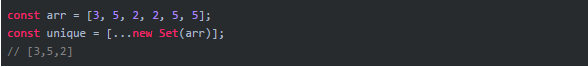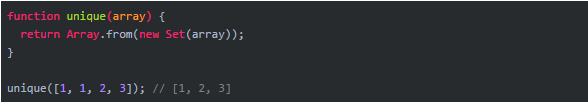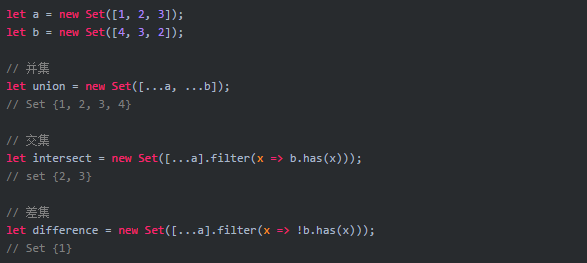# js中的new Set去重用法

js中的new Set去重用法

ES6 提供了新的数据结构 Set。它类似于数组，但是成员的值都是唯一的，没有重复的值。Set 本身是一个构造函数，用来生成 Set 数据结构。``````const arr = [3,5,2,2,5,5]
const unique = [...new Set(arr)]``````

Array.from 方法可以将 Set 结构转为数组。我们可以专门编写使用一个去重的函数``````function unique(arr){
return Array.from(new Set(arr))
}

unique([1,,1,2,2,3,3])````````````let a = new Set([1,2,3])
let b = new Set([4,3,2])

// 并集
let union = new Set([...a, ...b])

// 交集
let intersect = new Set([...a].filter(x => b.has(x)))

// 差集
let diff = new Set([...a].filter(x => !b.has(x)))
Set {1}``````

#### 评论

• 随机获取